Successfully reported this slideshow.
We use your LinkedIn profile and activity data to personalize ads and to show you more relevant ads. You can change your ad preferences anytime.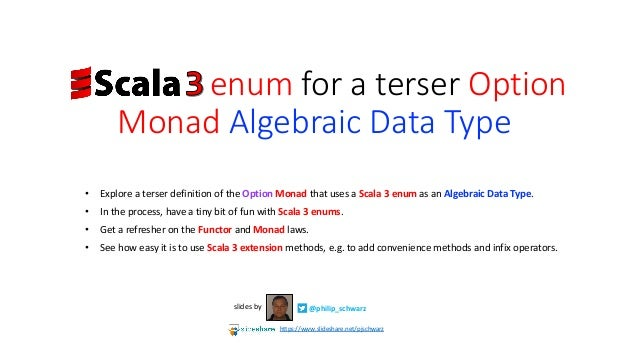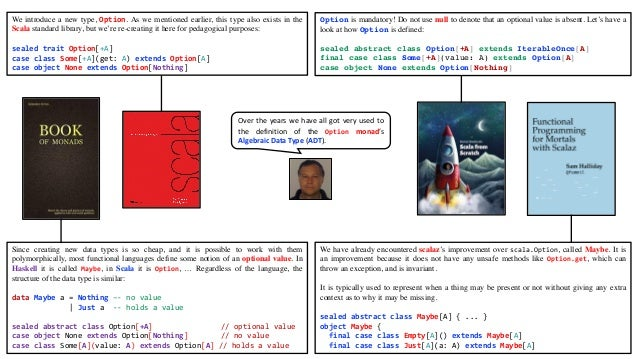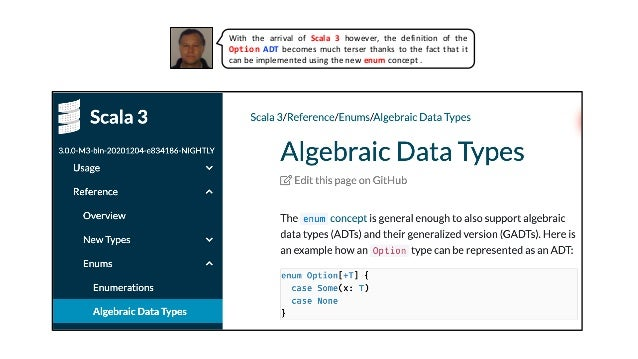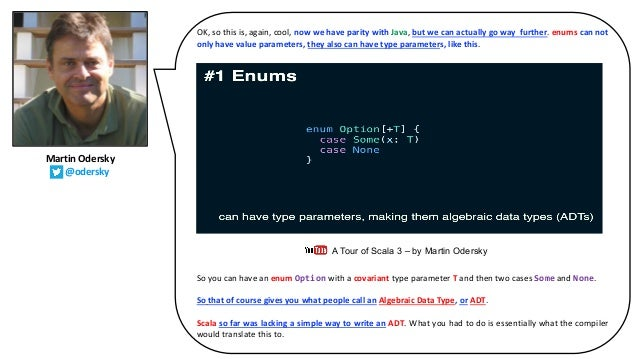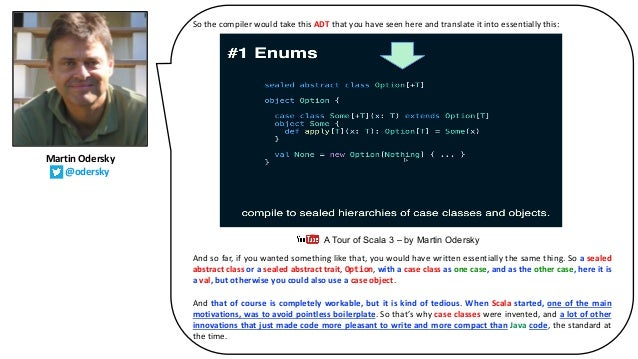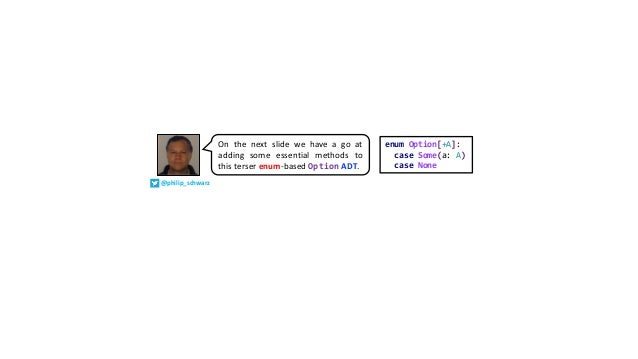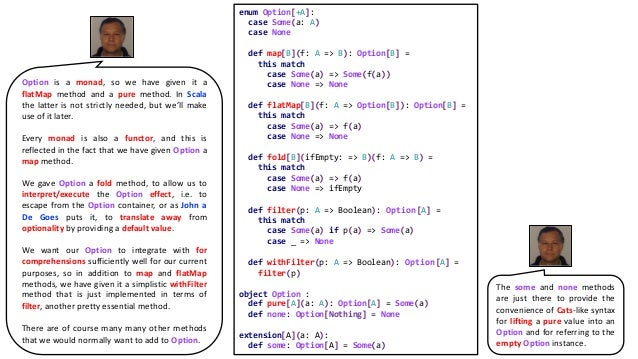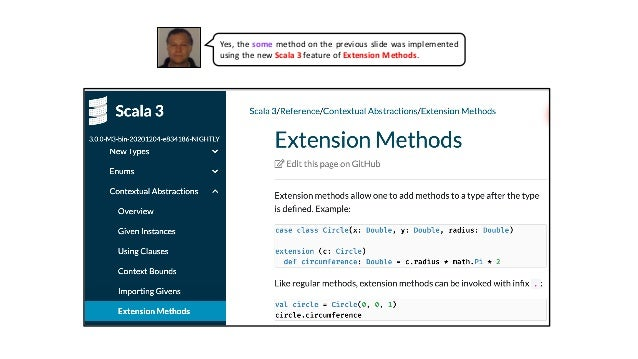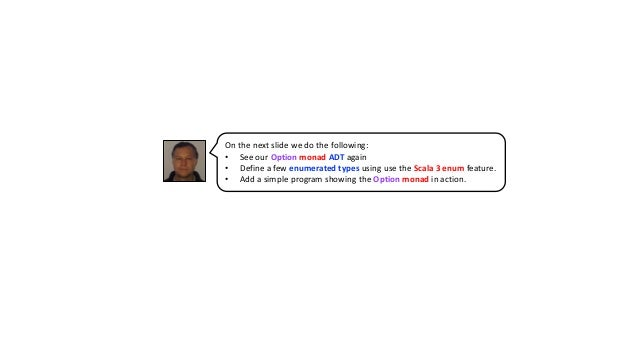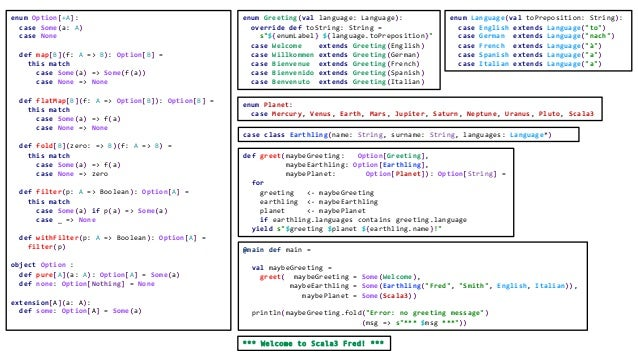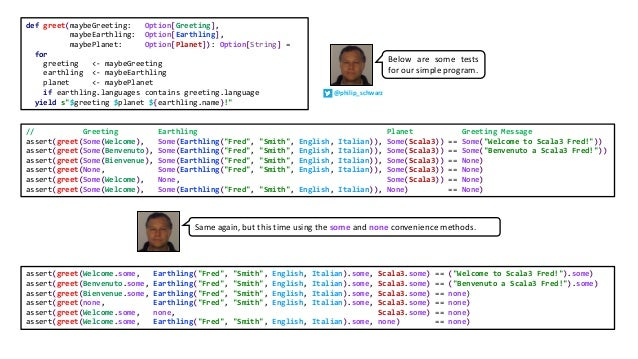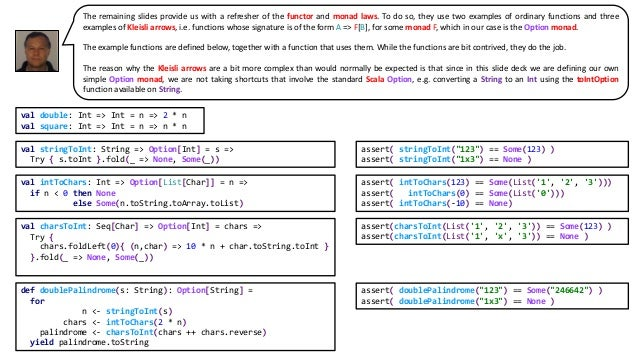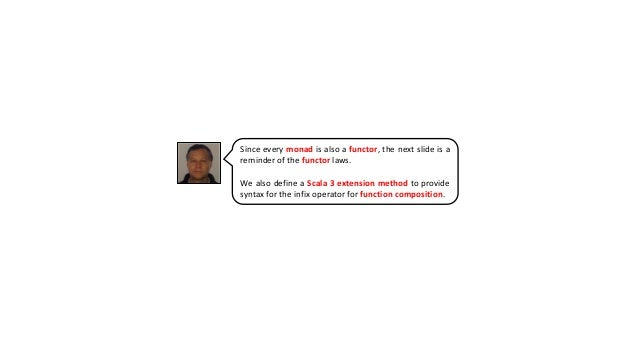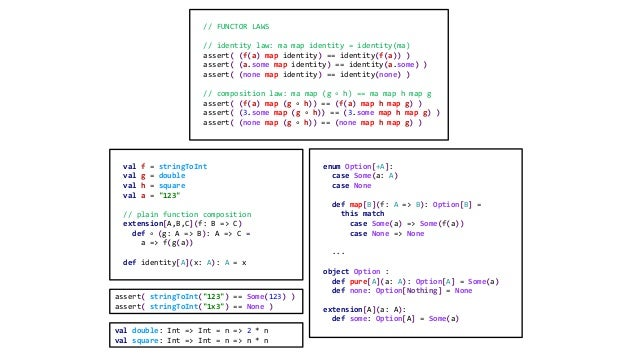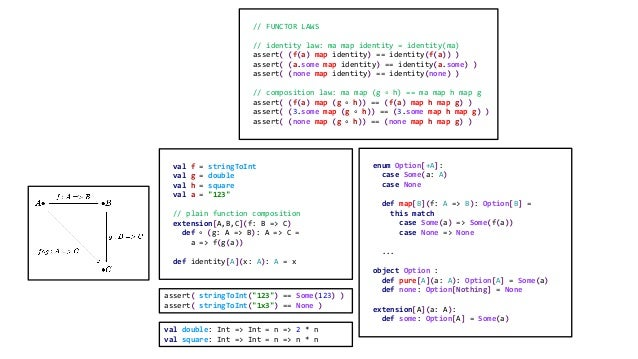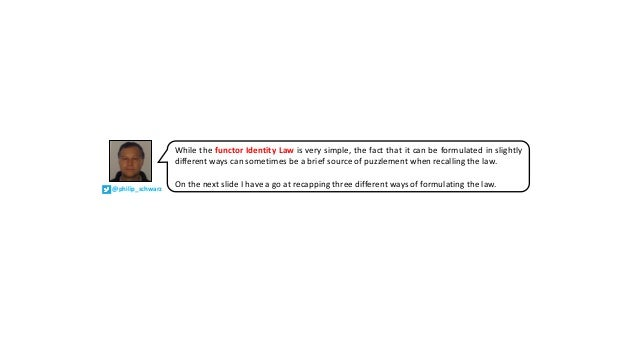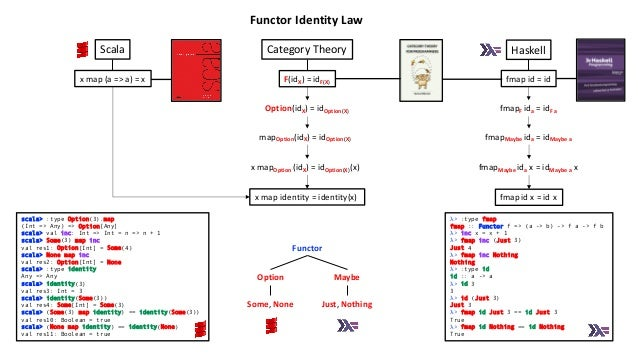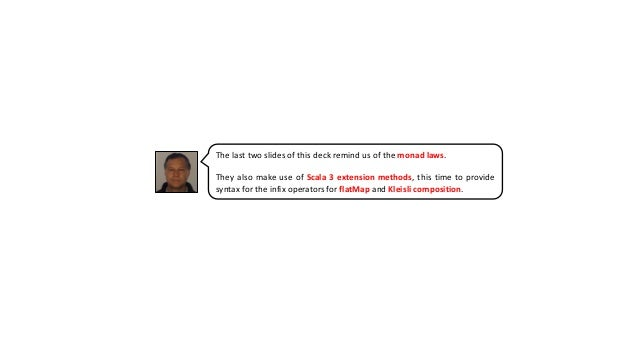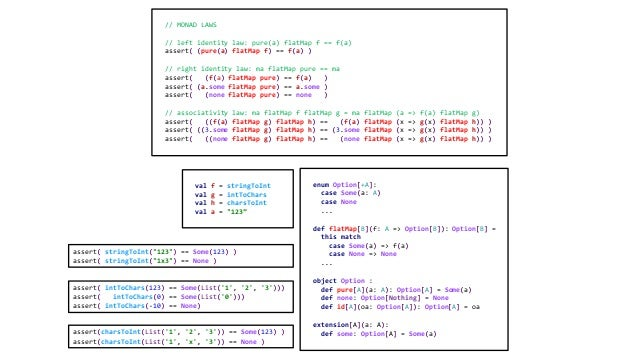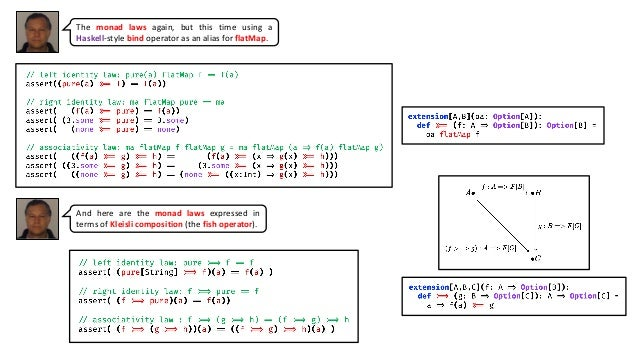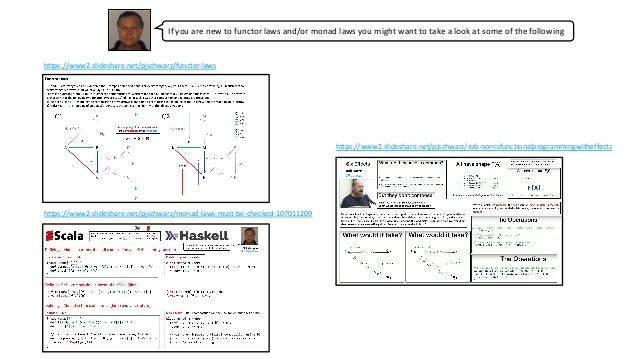Upcoming SlideShare
Loading in …5
×

# Scala 3 enum for a terser Option Monad Algebraic Data Type

(download for flawless slides)
* Explore a terser definition of the Option Monad that uses a Scala 3 enum as an Algebraic Data Type.
* In the process, have a tiny bit of fun with Scala 3 enums.
* Get a refresher on the Functor and Monad laws.
* See how easy it is to use Scala 3 extension methods, e.g. to add convenience methods and infix operators.

The diagrams for function composition and Kleisli composition were made using https://q.uiver.app/ by https://twitter.com/varkora.

Source code: https://github.com/philipschwarz/scala-3-enum-for-terser-option-monad-algebraic-data-type

Errata:
slide 14 is an unwanted leftover - it is the same as slide 15 minus a diagram.
on slide 19, the colons in the extension method declarations are not needed

• Full Name
Comment goes here.

Are you sure you want to Yes No
Your message goes here• Be the first to comment

### Scala 3 enum for a terser Option Monad Algebraic Data Type

1. 1. • Explore a terser definition of the Option Monad that uses a Scala 3 enum as an Algebraic Data Type. • In the process, have a tiny bit of fun with Scala 3 enums. • Get a refresher on the Functor and Monad laws. • See how easy it is to use Scala 3 extension methods, e.g. to add convenience methods and infix operators. @philip_schwarzslides by https://www.slideshare.net/pjschwarz enum for a terser Option Monad Algebraic Data Type..…
2. 2. We introduce a new type, Option. As we mentioned earlier, this type also exists in the Scala standard library, but we’re re-creating it here for pedagogical purposes: sealed trait Option[+A] case class Some[+A](get: A) extends Option[A] case object None extends Option[Nothing] Option is mandatory! Do not use null to denote that an optional value is absent. Let’s have a look at how Option is defined: sealed abstract class Option[+A] extends IterableOnce[A] final case class Some[+A](value: A) extends Option[A] case object None extends Option[Nothing] Since creating new data types is so cheap, and it is possible to work with them polymorphically, most functional languages define some notion of an optional value. In Haskell it is called Maybe, in Scala it is Option, … Regardless of the language, the structure of the data type is similar: data Maybe a = Nothing –- no value | Just a -- holds a value sealed abstract class Option[+A] // optional value case object None extends Option[Nothing] // no value case class Some[A](value: A) extends Option[A] // holds a value We have already encountered scalaz’s improvement over scala.Option, called Maybe. It is an improvement because it does not have any unsafe methods like Option.get, which can throw an exception, and is invariant. It is typically used to represent when a thing may be present or not without giving any extra context as to why it may be missing. sealed abstract class Maybe[A] { ... } object Maybe { final case class Empty[A]() extends Maybe[A] final case class Just[A](a: A) extends Maybe[A] Over the years we have all got very used to the definition of the Option monad’s Algebraic Data Type (ADT).
3. 3. With the arrival of Scala 3 however, the definition of the Option ADT becomes much terser thanks to the fact that it can be implemented using the new enum concept .
4. 4. OK, so this is, again, cool, now we have parity with Java, but we can actually go way further. enums can not only have value parameters, they also can have type parameters, like this. So you can have an enum Option with a covariant type parameter T and then two cases Some and None. So that of course gives you what people call an Algebraic Data Type, or ADT. Scala so far was lacking a simple way to write an ADT. What you had to do is essentially what the compiler would translate this to. A Tour of Scala 3 – by Martin Odersky Martin Odersky @odersky
5. 5. So the compiler would take this ADT that you have seen here and translate it into essentially this: And so far, if you wanted something like that, you would have written essentially the same thing. So a sealed abstract class or a sealed abstract trait, Option, with a case class as one case, and as the other case, here it is a val, but otherwise you could also use a case object. And that of course is completely workable, but it is kind of tedious. When Scala started, one of the main motivations, was to avoid pointless boilerplate. So that’s why case classes were invented, and a lot of other innovations that just made code more pleasant to write and more compact than Java code, the standard at the time. A Tour of Scala 3 – by Martin Odersky Martin Odersky @odersky
6. 6. enum Option[+A]: case Some(a: A) case None On the next slide we have a go at adding some essential methods to this terser enum-based Option ADT. @philip_schwarz
7. 7. enum Option[+A]: case Some(a: A) case None def map[B](f: A => B): Option[B] = this match case Some(a) => Some(f(a)) case None => None def flatMap[B](f: A => Option[B]): Option[B] = this match case Some(a) => f(a) case None => None def fold[B](ifEmpty: => B)(f: A => B) = this match case Some(a) => f(a) case None => ifEmpty def filter(p: A => Boolean): Option[A] = this match case Some(a) if p(a) => Some(a) case _ => None def withFilter(p: A => Boolean): Option[A] = filter(p) object Option : def pure[A](a: A): Option[A] = Some(a) def none: Option[Nothing] = None extension[A](a: A): def some: Option[A] = Some(a) Option is a monad, so we have given it a flatMap method and a pure method. In Scala the latter is not strictly needed, but we’ll make use of it later. Every monad is also a functor, and this is reflected in the fact that we have given Option a map method. We gave Option a fold method, to allow us to interpret/execute the Option effect, i.e. to escape from the Option container, or as John a De Goes puts it, to translate away from optionality by providing a default value. We want our Option to integrate with for comprehensions sufficiently well for our current purposes, so in addition to map and flatMap methods, we have given it a simplistic withFilter method that is just implemented in terms of filter, another pretty essential method. There are of course many many other methods that we would normally want to add to Option. The some and none methods are just there to provide the convenience of Cats-like syntax for lifting a pure value into an Option and for referring to the empty Option instance.
8. 8. Yes, the some method on the previous slide was implemented using the new Scala 3 feature of Extension Methods.
9. 9. On the next slide we do the following: • See our Option monad ADT again • Define a few enumerated types using use the Scala 3 enum feature. • Add a simple program showing the Option monad in action.
10. 10. enum Option[+A]: case Some(a: A) case None def map[B](f: A => B): Option[B] = this match case Some(a) => Some(f(a)) case None => None def flatMap[B](f: A => Option[B]): Option[B] = this match case Some(a) => f(a) case None => None def fold[B](zero: => B)(f: A => B) = this match case Some(a) => f(a) case None => zero def filter(p: A => Boolean): Option[A] = this match case Some(a) if p(a) => Some(a) case _ => None def withFilter(p: A => Boolean): Option[A] = filter(p) object Option : def pure[A](a: A): Option[A] = Some(a) def none: Option[Nothing] = None extension[A](a: A): def some: Option[A] = Some(a) enum Greeting(val language: Language): override def toString: String = s"\${enumLabel} \${language.toPreposition}" case Welcome extends Greeting(English) case Willkommen extends Greeting(German) case Bienvenue extends Greeting(French) case Bienvenido extends Greeting(Spanish) case Benvenuto extends Greeting(Italian) enum Language(val toPreposition: String): case English extends Language("to") case German extends Language("nach") case French extends Language("à") case Spanish extends Language("a") case Italian extends Language("a") enum Planet: case Mercury, Venus, Earth, Mars, Jupiter, Saturn, Neptune, Uranus, Pluto, Scala3 case class Earthling(name: String, surname: String, languages: Language*) def greet(maybeGreeting: Option[Greeting], maybeEarthling: Option[Earthling], maybePlanet: Option[Planet]): Option[String] = for greeting <- maybeGreeting earthling <- maybeEarthling planet <- maybePlanet if earthling.languages contains greeting.language yield s"\$greeting \$planet \${earthling.name}!" @main def main = val maybeGreeting = greet( maybeGreeting = Some(Welcome), maybeEarthling = Some(Earthling("Fred", "Smith", English, Italian)), maybePlanet = Some(Scala3)) println(maybeGreeting.fold("Error: no greeting message") (msg => s"*** \$msg ***")) *** Welcome to Scala3 Fred! ***
11. 11. def greet(maybeGreeting: Option[Greeting], maybeEarthling: Option[Earthling], maybePlanet: Option[Planet]): Option[String] = for greeting <- maybeGreeting earthling <- maybeEarthling planet <- maybePlanet if earthling.languages contains greeting.language yield s"\$greeting \$planet \${earthling.name}!" // Greeting Earthling Planet Greeting Message assert(greet(Some(Welcome), Some(Earthling("Fred", "Smith", English, Italian)), Some(Scala3)) == Some("Welcome to Scala3 Fred!")) assert(greet(Some(Benvenuto), Some(Earthling("Fred", "Smith", English, Italian)), Some(Scala3)) == Some("Benvenuto a Scala3 Fred!")) assert(greet(Some(Bienvenue), Some(Earthling("Fred", "Smith", English, Italian)), Some(Scala3)) == None) assert(greet(None, Some(Earthling("Fred", "Smith", English, Italian)), Some(Scala3)) == None) assert(greet(Some(Welcome), None, Some(Scala3)) == None) assert(greet(Some(Welcome), Some(Earthling("Fred", "Smith", English, Italian)), None) == None) assert(greet(Welcome.some, Earthling("Fred", "Smith", English, Italian).some, Scala3.some) == ("Welcome to Scala3 Fred!").some) assert(greet(Benvenuto.some, Earthling("Fred", "Smith", English, Italian).some, Scala3.some) == ("Benvenuto a Scala3 Fred!").some) assert(greet(Bienvenue.some, Earthling("Fred", "Smith", English, Italian).some, Scala3.some) == none) assert(greet(none, Earthling("Fred", "Smith", English, Italian).some, Scala3.some) == none) assert(greet(Welcome.some, none, Scala3.some) == none) assert(greet(Welcome.some, Earthling("Fred", "Smith", English, Italian).some, none) == none) Same again, but this time using the some and none convenience methods. Below are some tests for our simple program. @philip_schwarz
12. 12. val stringToInt: String => Option[Int] = s => Try { s.toInt }.fold(_ => None, Some(_)) assert( stringToInt("123") == Some(123) ) assert( stringToInt("1x3") == None ) assert( intToChars(123) == Some(List('1', '2', '3'))) assert( intToChars(0) == Some(List('0'))) assert( intToChars(-10) == None) assert(charsToInt(List('1', '2', '3')) == Some(123) ) assert(charsToInt(List('1', 'x', '3')) == None ) val intToChars: Int => Option[List[Char]] = n => if n < 0 then None else Some(n.toString.toArray.toList) val charsToInt: Seq[Char] => Option[Int] = chars => Try { chars.foldLeft(0){ (n,char) => 10 * n + char.toString.toInt } }.fold(_ => None, Some(_)) def doublePalindrome(s: String): Option[String] = for n <- stringToInt(s) chars <- intToChars(2 * n) palindrome <- charsToInt(chars ++ chars.reverse) yield palindrome.toString assert( doublePalindrome("123") == Some("246642") ) assert( doublePalindrome("1x3") == None ) The remaining slides provide us with a refresher of the functor and monad laws. To do so, they use two examples of ordinary functions and three examples of Kleisli arrows, i.e. functions whose signature is of the form A => F[B], for some monad F, which in our case is the Option monad. The example functions are defined below, together with a function that uses them. While the functions are bit contrived, they do the job. The reason why the Kleisli arrows are a bit more complex than would normally be expected is that since in this slide deck we are defining our own simple Option monad, we are not taking shortcuts that involve the standard Scala Option, e.g. converting a String to an Int using the toIntOption function available on String. val double: Int => Int = n => 2 * n val square: Int => Int = n => n * n
13. 13. Since every monad is also a functor, the next slide is a reminder of the functor laws. We also define a Scala 3 extension method to provide syntax for the infix operator for function composition.
14. 14. // FUNCTOR LAWS // identity law: ma map identity = identity(ma) assert( (f(a) map identity) == identity(f(a)) ) assert( (a.some map identity) == identity(a.some) ) assert( (none map identity) == identity(none) ) // composition law: ma map (g ∘ h) == ma map h map g assert( (f(a) map (g ∘ h)) == (f(a) map h map g) ) assert( (3.some map (g ∘ h)) == (3.some map h map g) ) assert( (none map (g ∘ h)) == (none map h map g) ) val f = stringToInt val g = double val h = square val a = "123" // plain function composition extension[A,B,C](f: B => C) def ∘ (g: A => B): A => C = a => f(g(a)) def identity[A](x: A): A = x enum Option[+A]: case Some(a: A) case None def map[B](f: A => B): Option[B] = this match case Some(a) => Some(f(a)) case None => None ... object Option : def pure[A](a: A): Option[A] = Some(a) def none: Option[Nothing] = None extension[A](a: A): def some: Option[A] = Some(a) assert( stringToInt("123") == Some(123) ) assert( stringToInt("1x3") == None ) val double: Int => Int = n => 2 * n val square: Int => Int = n => n * n
15. 15. // FUNCTOR LAWS // identity law: ma map identity = identity(ma) assert( (f(a) map identity) == identity(f(a)) ) assert( (a.some map identity) == identity(a.some) ) assert( (none map identity) == identity(none) ) // composition law: ma map (g ∘ h) == ma map h map g assert( (f(a) map (g ∘ h)) == (f(a) map h map g) ) assert( (3.some map (g ∘ h)) == (3.some map h map g) ) assert( (none map (g ∘ h)) == (none map h map g) ) val f = stringToInt val g = double val h = square val a = "123" // plain function composition extension[A,B,C](f: B => C) def ∘ (g: A => B): A => C = a => f(g(a)) def identity[A](x: A): A = x enum Option[+A]: case Some(a: A) case None def map[B](f: A => B): Option[B] = this match case Some(a) => Some(f(a)) case None => None ... object Option : def pure[A](a: A): Option[A] = Some(a) def none: Option[Nothing] = None extension[A](a: A): def some: Option[A] = Some(a) assert( stringToInt("123") == Some(123) ) assert( stringToInt("1x3") == None ) val double: Int => Int = n => 2 * n val square: Int => Int = n => n * n
16. 16. @philip_schwarz While the functor Identity Law is very simple, the fact that it can be formulated in slightly different ways can sometimes be a brief source of puzzlement when recalling the law. On the next slide I have a go at recapping three different ways of formulating the law.
17. 17. F(idX) = idF(X) fmap id = id fmapMaybe ida x = idMaybe a x Option(idX) = idOption(X) x map (a => a) = x mapOption(idX) = idOption(X) x mapOption (idX) = idOption(X)(x) fmapF ida = idF a fmapMaybe ida = idMaybe a λ> :type fmap fmap :: Functor f => (a -> b) -> f a -> f b λ> inc x = x + 1 λ> fmap inc (Just 3) Just 4 λ> fmap inc Nothing Nothing λ> :type id id :: a -> a λ> id 3 3 λ> id (Just 3) Just 3 λ> fmap id Just 3 == id Just 3 True λ> fmap id Nothing == id Nothing True scala> :type Option(3).map (Int => Any) => Option[Any] scala> val inc: Int => Int = n => n + 1 scala> Some(3) map inc val res1: Option[Int] = Some(4) scala> None map inc val res2: Option[Int] = None scala> :type identity Any => Any scala> identity(3) val res3: Int = 3 scala> identity(Some(3)) val res4: Some[Int] = Some(3) scala> (Some(3) map identity) == identity(Some(3)) val res10: Boolean = true scala> (None map identity) == identity(None) val res11: Boolean = true Functor Option Maybe x map identity = identity(x) fmap id x = id x Category TheoryScala Haskell Some, None Just, Nothing Functor Identity Law
18. 18. The last two slides of this deck remind us of the monad laws. They also make use of Scala 3 extension methods, this time to provide syntax for the infix operators for flatMap and Kleisli composition.
19. 19. // MONAD LAWS // left identity law: pure(a) flatMap f == f(a) assert( (pure(a) flatMap f) == f(a) ) // right identity law: ma flatMap pure == ma assert( (f(a) flatMap pure) == f(a) ) assert( (a.some flatMap pure) == a.some ) assert( (none flatMap pure) == none ) // associativity law: ma flatMap f flatMap g = ma flatMap (a => f(a) flatMap g) assert( ((f(a) flatMap g) flatMap h) == (f(a) flatMap (x => g(x) flatMap h)) ) assert( ((3.some flatMap g) flatMap h) == (3.some flatMap (x => g(x) flatMap h)) ) assert( ((none flatMap g) flatMap h) == (none flatMap (x => g(x) flatMap h)) ) enum Option[+A]: case Some(a: A) case None ... def flatMap[B](f: A => Option[B]): Option[B] = this match case Some(a) => f(a) case None => None ... object Option : def pure[A](a: A): Option[A] = Some(a) def none: Option[Nothing] = None def id[A](oa: Option[A]): Option[A] = oa extension[A](a: A): def some: Option[A] = Some(a) val f = stringToInt val g = intToChars val h = charsToInt val a = "123” assert( stringToInt("123") == Some(123) ) assert( stringToInt("1x3") == None ) assert( intToChars(123) == Some(List('1', '2', '3'))) assert( intToChars(0) == Some(List('0'))) assert( intToChars(-10) == None) assert(charsToInt(List('1', '2', '3')) == Some(123) ) assert(charsToInt(List('1', 'x', '3')) == None )
20. 20. The monad laws again, but this time using a Haskell-style bind operator as an alias for flatMap. And here are the monad laws expressed in terms of Kleisli composition (the fish operator).
21. 21. If you are new to functor laws and/or monad laws you might want to take a look at some of the following https://www2.slideshare.net/pjschwarz/functor-laws https://www2.slideshare.net/pjschwarz/monad-laws-must-be-checked-107011209 https://www2.slideshare.net/pjschwarz/rob-norrisfunctionalprogrammingwitheffects
22. 22. That’s all. I hope you found it useful. @philip_schwarz